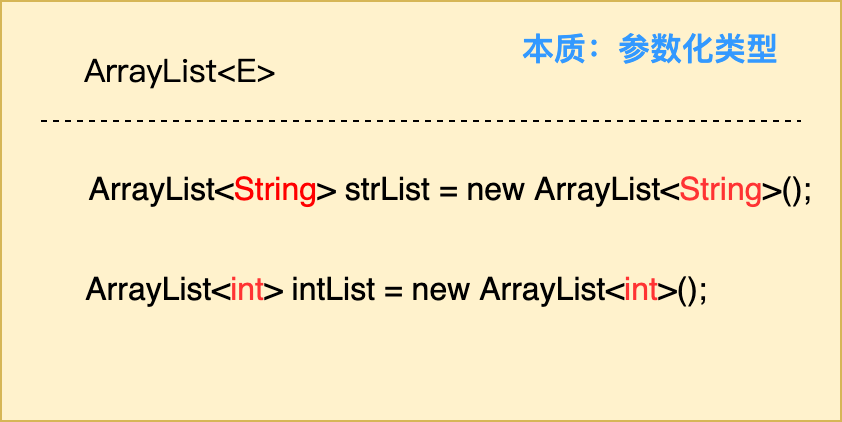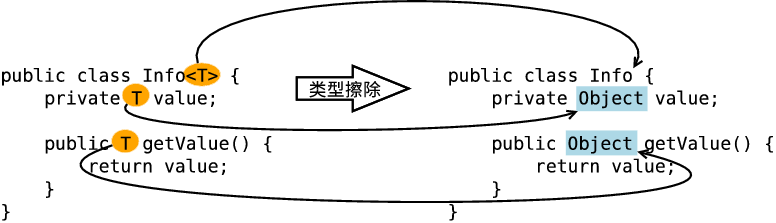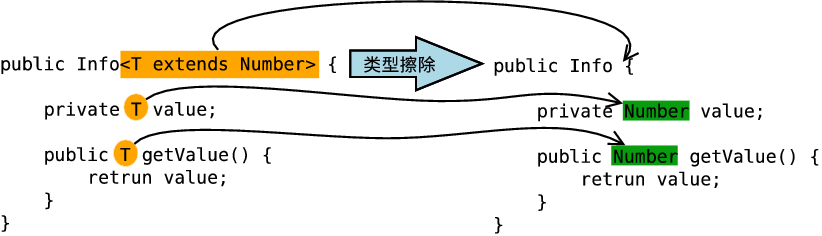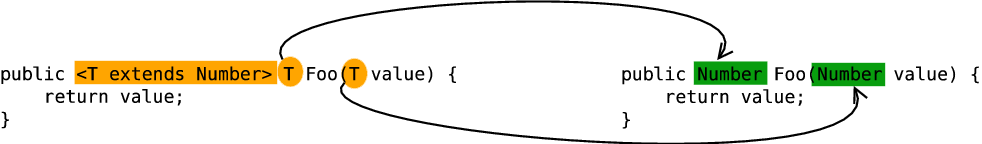# Java核心知识1：泛型机制详解# 1 理解泛型的本质

JDK 1.5开始引入Java泛型（generics）这个特性，该特性提供了编译时类型安全检测机制，允许程序员在编译时检测到非法的类型。# 2 泛型的作用## 2.1 泛型如何保证类型安全

``````public static void noGenericTest() {
// 编译正常通过，但是使用的时候可能转换处理出现问题
ArrayList arr = new ArrayList();
}
``````

``````public static void genericTest() {
// 编译不通过，直接提示异常，Required type：String
ArrayList<String> arr = new ArrayList<>();
}
``````

## 2.2 类型自动转换，消除强转

``````ArrayList list  = new ArrayList();
int i = (int) list.get(0);  // 需强转
``````

``````ArrayList<Integer> list  = new ArrayList<>();
int i = list.get(0);  // 无需转换
``````

## 2.3 避免装箱、拆箱，提高性能

``````object a=1;//由于是object类型，会自动进行装箱操作。

int b=(int)a;//强制转换，拆箱操作。这样一去一来，当次数多了以后会影响程序的运行效率。

``````

``````public static T GetValue<T>(T a) {
return a;
}

public static void Main(){
int b=GetValue<int>(1);//使用这个方法的时候已经指定了类型是int，所以不会有装箱和拆箱的操作。
}
``````

## 2.4 提升程序可复用性

``````private static int add(int a, int b) {
System.out.println(a + "+" + b + "=" + (a + b));
return a + b;
}

private static float add(float a, float b) {
System.out.println(a + "+" + b + "=" + (a + b));
return a + b;
}

private static double add(double a, double b) {
System.out.println(a + "+" + b + "=" + (a + b));
return a + b;
}
``````

``````private static <T extends Number> double add(T a, T b) {
System.out.println(a + "+" + b + "=" + (a.doubleValue() + b.doubleValue()));
return a.doubleValue() + b.doubleValue();
}
``````

# 3 泛型的使用

## 3.1 泛型类

``````public class 类名 <泛型类型1,...> {
// todo
}
``````

``````public class GenericClass<ab,a,c> {
// todo
}
``````

T：任意类型 type
E：集合中元素的类型 element
K：key-value形式 key
V： key-value形式 value
N： Number（数值类型）
？： 表示不确定的java类型

``````@Data
public class Response<T> {
/**
* 状态
*/
private boolean status;
/**
* 编码
*/
private Integer code;
/**
* 消息
*/
private String msg;
/**
* 接口返回内容，不同的接口返回的内容不一致，使用泛型数据
*/
private T data;

/**
* 构造
* @param status
* @param code
* @param msg
* @param data
*/
public Response(boolean status,int code,String msg,T data) {
this.status = status;
this.code = code;
this.msg = msg;
this.data = data;
}
}
``````

``````@Data
public class UserInfo {
/**
* 用户编号
*/
private String userCode;
/**
* 用户名称
*/
}
``````

``````        /**
* 返回字符串
*/
Response<String> responseStr = new Response<>(true,200,"success","Hello Word");

/**
* 返回用户对象
*/
UserInfo userInfo = new UserInfo();
userInfo.setUserCode("123456");
Response<UserInfo> responseObj = new Response<>(true,200,"success",userInfo);
``````

``````{
"status": true,
"code": 200,
"msg": "success",
"data": "Hello Word"
}
// 和
{
"status": true,
"code": 200,
"msg": "success",
"data": {
"user_code": "123456",
"user_name": "Brand"
}
}
``````

## 3.2 泛型接口

``````public interface 接口名<T> {
// todo
}
``````

``````public interface GenericInterface<T> {
void show(T value);}
}
public class StringShowImpl implements GenericInterface<String> {
@Override
public void show(String value) {
System.out.println(value);
}}

public class NumberShowImpl implements GenericInterface<Integer> {
@Override
public void show(Integer value) {
System.out.println(value);
}}

``````

``````// 编译的时候会报错，因为前后类型不一致
GenericInterface<String> genericInterface = new NumberShowImpl();
// 编译正常，前面泛型接口不指定类型，由new后面的实例化来推导。
GenericInterface g1 = new NumberShowImpl();
GenericInterface g2 = new StringShowImpl();
``````

## 3.3 泛型方法

``````public <泛型类型> 返回类型 方法名（泛型类型 变量名） {
// todo
}
``````

``````/**
* 泛型方法
* @param <T> 泛型的类型
* @param c 传入泛型的参数对象
* @return T 返回值为T类型
* 说明：
*   1）public 与 返回值中间<T>非常重要，可以理解为声明此方法为泛型方法。
*   2）只有声明了<T>的方法才是泛型方法，泛型类中的使用了泛型的成员方法并不是泛型方法。
*   3）<T>表明该方法将使用泛型类型T，此时才可以在方法中使用泛型类型T。
*   4）与泛型类的定义一样，此处T可以随便写为任意标识，常见的如T、E等形式的参数常用于表示泛型。
*/
public <T> T genercMethod(T c) {
System.out.println(c.getClass());
System.out.println(c);
return c;
}

public static void main(String[] args) {
GenericsClassDemo<String> genericString  = new GenericsClassDemo("Hello World"); //这里的泛型跟下面调用的泛型方法可以不一样。
String str = genericString.genercMethod("brand");//传入的是String类型,返回的也是String类型
Integer i = genericString.genercMethod(100);//传入的是Integer类型,返回的也是Integer类型
}
``````

``````class java.lang.String
brand

class java.lang.Integer
100
``````

## 3.4 泛型通配符（上下界）

Java泛型的通配符是用于解决泛型之间引用传递问题的特殊语法, 主要有以下三类:

• 无边界的通配符，使用精确的参数类型
• 关键字声明了类型的上界，表示参数化的类型可能是所指定的类型，或者是此类型的子类
• 关键字声明了类型的下界，表示参数化的类型可能是指定的类型，或者是此类型的父类

``````// 表示类型参数可以是任何类型
public class B<?> {
}

// 上界：表示类型参数必须是A或者是A的子类
public class B<T extends A> {
}

// 下界：表示类型参数必须是A或者是A的超类型
public class B<T supers A> {
}
``````

``````class Info<T extends Number>{    // 此处泛型只能是数字类型
private T var ;        // 定义泛型变量
public void setVar(T var){
this.var = var ;
}
public T getVar(){
return this.var ;
}
public String toString(){    // 直接打印
return this.var.toString() ;
}
}
public class demo1{
public static void main(String args[]){
Info<Integer> i1 = new Info<Integer>() ;        // 声明Integer的泛型对象
}
}
``````

``````class Info<T>{
private T var ;        // 定义泛型变量
public void setVar(T var){
this.var = var ;
}
public T getVar(){
return this.var ;
}
public String toString(){    // 直接打印
return this.var.toString() ;
}
}
public class GenericsDemo21{
public static void main(String args[]){
Info<String> i1 = new Info<String>() ;        // 声明String的泛型对象
Info<Object> i2 = new Info<Object>() ;        // 声明Object的泛型对象
i1.setVar("hello") ;
i2.setVar(new Object()) ;
fun(i1) ;
fun(i2) ;
}
public static void fun(Info<? super String> temp){    // 只能接收String或Object类型的泛型，String类的父类只有Object类
System.out.print(temp + ", ") ;
}
}
``````

# 4 泛型实现原理

Java泛型这个特性是从JDK 1.5才开始加入的，因此为了兼容之前的版本，Java泛型的实现采取了“伪泛型”的策略，即Java在语法上支持泛型，但是在编译阶段会进行所谓的“类型擦除”（Type Erasure），

## 4.1 泛型的类型擦除原则

• 消除类型参数声明，即删除<>及其包围的部分。
• 根据类型参数的上下界推断并替换所有的类型参数为原生态类型：如果类型参数是无限制通配符或没有上下界限定则替换为Object，如果存在上下界限定则根据子类替换原则取类型参数的最左边限定类型（即父类）。
• 为了保证类型安全，必要时插入强制类型转换代码。
• 自动产生“桥接方法”以保证擦除类型后的代码仍然具有泛型的“多态性”。

## 4.2 擦除的方式2 total views,  1 views today